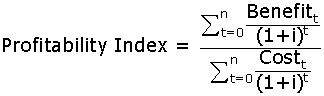# Profitability Index

Profitability ratios Print Email

The profitability index (PI) refers to the ratio of discounted benefits over the discounted costs. It is an evaluation of the profitability of an investment and can be compared with the profitability of other similar investments which are under consideration. the profitability index is also referred to as benefit-cost ratio, cost-benefit ratio, or even capital rationing. The profitability index is one of the numerous ways used to quantify and measure the efficiency of a proposed investment.

Calculation (formula) of Profitability Index

The formula used for calculating the Profitability index is:i = the interest rate per period (discount rate).
n = the number of periods.

Where the numerator shows the discounted sum of benefits and the denominator represents the discounted sum of costs related with a particular project. This equation can, however, also be used to infer the Internal Rate of Return (IRR) which arises when Profitability Index equals 1.

Interpretation of Profitability Index

An investment project or proposal is considered to be profitable if it features a profitability index above 1. For example, a profitability index of 0.89 indicates that the project or investment will not make us any profits. On the contrary, a profitability index equal to 1 indicates a break even on the investments without making any profits.

The advantages of profitability index for a firm are listed below:

• The profitability index tells about an investment increasing or decreasing the firm’s value

• The profitability index takes into consideration all cash flows of the project.

• The profitability index takes the time value of money into consideration.

• The profitability index also considers the risk involved in future cash flows with the help of cost of capital.

• The profitability index is also helpful in ranking and picking projects while rationing of capital.

In addition to the aforesaid advantages, there are also certain disadvantages featured by the profitability index. These include:

• An estimate about the cost of capital is required so as to calculate the profitability index of a firm.

• The profitability index of a firm might not, sometimes, provide the correct decision while being used to compare mutually exclusive projects under consideration.